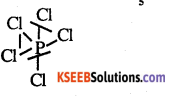# 2nd PUC Chemistry Question Bank Chapter 7 The p-Block Elements

## Karnataka 2nd PUC Chemistry Question Bank Chapter 7 The p-Block Elements

### 2nd PUC Chemistry The p-Block Elements NCERT Textbook Questions and Answers

Question 1.
Discuss the general characteristics of Group 15 elements with reference to their electronic configuration, oxidation state, atomic size, ionisation enthalpy and electronegativity.
(i) Electronic configuration ‘All the elements in group 15 have 5 valence electrons. Their general electronic configuration is ns2np3

(ii) Oxidation states
All these elements have 5 valence electrons and require three more electrons to complete octets. However gaining electrons is very difficult as the nucleus will have to attract three more electrons. This can take place only with nitrogen as it is the smallest in size and the distance between the nucleus and the valance shell is relatively small. The remaining elements of this group show a formal oxidation state of -3 in their covalent compounds.

In addition to – 3 state, N and P also show -1 and.-2 oxidation states. All the elements present in this group show +3 and +5 oxidation states. However stability of +5 oxidation state decreases down the group, whereas the stability of+3 oxidation state increases. This happens because of the inert pair effect.

(iii) Ionization energy and electronegativity First ionization energy decreases on moving down a group. This is because of increasing atomic sizes. As we move down a group, electronegativity decreases, owing to an increase in size.

(iv) Atomic size
On moving down a group, the atomic size increases. This increase in the atomic size is attributed to an increase in number of shell.

Question 2
Why does the reactivity of nitrogen differ
from phosphorus? i
Nitrogen is chemically less reactive. This is because of high stability of its molecule, N2. In N2 the two nitrogen atoms form a triple bond. This triple bond has a very high bond strength, which is very difficult to break. It is because of nitrogens small size that it is able to form pπ-pπ bonds with itself. This property is not exhibited by atoms such as phosphorus. Thus, phosphorus is more reactive than nitrogen.

Question 3
Discuss the trends in chemical reactivity of group 15 elements.
General trends in chemical properties of group-15
(i) Reactivity towards hydrogen:
The elements of group 15 react with hydrogen to form hydrides of type EH3, where E – N, P, As, Sb, or Bi. The stability of hydrides decreases on moving down from NH3 to BiH3

(ii) Reactivity towards oxygen:
The elements of group 15 form two types of oxides: E2O3 arid E2O5, where E = N, P, As, Sb or Bi. The oxide with the element in higher oxidation state is more acidic than the other. However, the acidic character decreases on moving down a group.

(iii) Reactivity towards halogens:
The group 15 element react with halogens to form two series of salts: EX3 and EX5. However, nitrogen does not form NX5 as H lacks the d-orbital. All trihalides (except NX3) are stable.

(iv) Reactivity towards metals:
The group 15 elements react with metals to form binary compounds in which these metals exhibit-3 oxidation states.

Question 4
Why does NH3 form hydrogen bond but PH3 does not?
Nitrogen is highly electronegative as compared to phosphorus. This causes a greater attraction of electrons towards nitrogen in NH3 than towards phosphorus in PH3. Hence, the extent of hydrogen bonding in PH3 is very less as compared to NH3.Question 5.
How is nitrogen prepared in the laboratory? Write the chemical equations of the reactions involved.
An aqueous solution of ammonium chloride is treated with sodium nitrite
NH4Cl + NaNO2(aq) → N2(g) + 2H2O(e) + NaCl(aq)
NO and HNO3 are produced in small amounts. These are impurities that can be removed on passing nitrogen gas through aqueous H2SO4 containing potassium dichromate

Question 6
How is ammonia manufactured industrially?
Ammonia is prepared on a large-scale by the Haber’s proceed.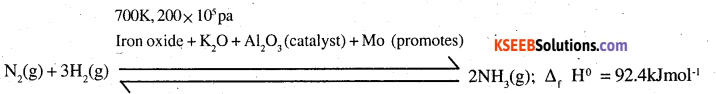Question 7
Illustrate how copper metal can give different products on reaction with HNO3.
On heating with dil HNO3, copper gives copper nitrate and nitric oxide.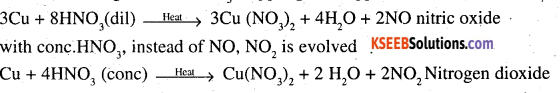Question 8
Give the resonating structures of NO2 and N2O5.
Resonating structures of NO2 are: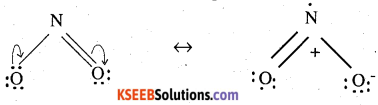Resonating structures of N2O5 are: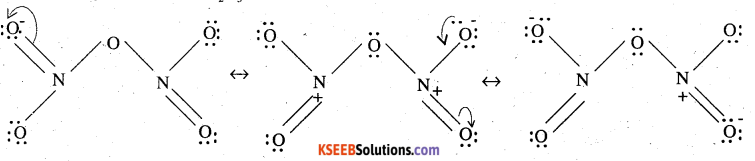Question 9.
The HNH angle value is higher than HPH, HAsH and HSbH angles. Why?
[Hint: Can be explained on the basis of sp3 hybridisation in NH3 and only s-p bonding between hydrogen and other elements of the group].
Hydride NH3, PH3, AsH3, SbH3
H-M-H angle 107°, 92°, 91°, 90°

The above trend in H-M-H bond angle can be explained on the basis of the electronegativity tif central atom. Since nitrogen is highly electronegative, there is high electron density around nitrogen. This causes greater repulsion between the electron pairs around nitrogen, resulting in maximum bond angle. We know that electronegativity decreases on moving down a group consequently. The repulsive interactions between the electron pairs decreases, thereby decreasing the HMH bond angle.Question 10.
Why does R3P = O exist but R3N = O does not (R = alkyl group)?
N (unlike p) lacks d-orbital. This restricts nitrogen to expand its coordination number beyond four. Hence, R3N = O doesnot exits.

Question 11.
Explain why NH3is basic while BiH3 is only feebly basic.
NH3 is distinctly basic while BiH3 is feebly basic.
Nitrogen has a small size due to which the lone pair of electrons is concentrated in a small region. This means that the charge density per unit volume is high. On moving down a group, the size of the central atom increases and the charge gets distributed over a large area decreasing the electron density. Hence the electron donating capacity of group 15 elements hydrides decreases on moving down the group.

Question 12.
Nitrogen exists as diatomic molecule and phosphorus as P4. Why?
Nitrogen owing to its small size has a tendency to form pn-pn multiple bonds with itself. Nitrogen thus forms a very stable diatomic molecule, N2. On moving down a group, the tendency to form pπ-pπ bonds decreases (because of the large size of heavier elements). Therefore, phosphorus (like other heavier metals) exists in the P4 state.

Question 13
Write main differences between the properties of white phosphorus and red phosphorus.

 White phosphorous Red phosphorous It is a soft and waxy solid. It possesses agarlic smell. It is hard and crystalline solid. It is poisonous without any smell It is insoluble in water but soluble in carbon disulphide It is non-poisonous It is insoluble in both water and carbon It undergoes spontaneous combustion in air disulphide It is relatively In both solid and vapour states, it exists as a P4 molecule less reactive It exists as a chain of tetrahedral P4.

Question 14
Why does nitrogen show catenation properties less than phosphorus?
The property of catenation depends upon the strength of the element bond. Since the N- N (159 kJ mol-1) bond strength is much weaker than P-P (213 kJ mol-1) bond strength, therefore nitrogen show less catenation properties than phosphorus.

Question 15
Give the disproportionation reaction of
H3PO3.
H3PO3 on heating undergoes self oxidation reduction, i.e. disproportionation to form PH, in which P is reduced and H3PO4 in which P is oxidised.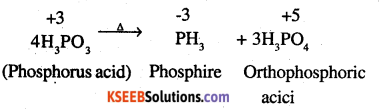Question 16.
Can PCl5 act as an oxidising as well as a reducing agent? Justify.
PCl5 can only act as an oxidizing agent. The highest oxidation state that P can show is +5. In PCl5 , phosphorus is in its highest oxidation state (+5). However it can decrease its oxidation state and act as an oxidizing agent.

Question 17.
Justify the placement of Q, S, Se, Te and Po in the same group of the periodic table in terms of electronic configuration, oxidation state and hydride formation.
The elements of group 16 are collectively called chalcogens.
(i) Elements of group 16 have six valence electrons each. The general electronic configuration of these elements is ns2 np4, where n varies from 2 to 6.
(ii) oxidation state:
As these elements have six valence electrons (ns2 np4). They should display an oxidation state of -2. However only oxygen predominantly shows the oxidation state of -2 owing to its high electronegativity. It also exhibits the oxidation . state of -1 (H2O2), Zero (O2), and +2 (OF2) However, the stability of the -2 oxidation state decreases on moving down a group due to a decrease in the electronegativity of the elements. The heavier elements of the group show an oxidation state of +2, +4 and +6 due to the availability of d-orbitals.

(iii) Formation of hydrides
These elements form hydrides of formula H2E, where E = O, S, Se, Te, PO. Oxygen and sulphur also form hydrides of type H2E2. These hydrides are quite volatile in nature.Question 18.
Why is dioxygen a gas but sulphur a solid?
Oxygen is smaller in size as compared to sulphur. Due to its smaller size, it can effectively form pπ-pπ bonds and form O2 (O = O) molecule. Also, the intermolecular forces in oxygen are weak Van dar Wall’s which cause
it to exist as gas. On the other hand, Sulphur does not form M2 molecule but exists as a puckered structure held to gather by strong covalent bonds. Hence it is a solid.

Question 19
Knowing the electron gain enthalpy . values for O → O and O → O2- as -141 and 702 kj mol-1 respectively, how can you account for the formation of a large number of oxides having O2- species and not O?
(Hint: Consider lattice energy factor in the formation of compounds).
Stability of an ionic compound depends on its lattice energy. More the lattice energy of a compound, more stable it will be. Lattice energy is directly proportional to the charge carried by an ion. When a metal combines with oxygen, the lattice energy of the oxide involving O2- ion is much more than the oxide involving O ion. Hence, we can say that formation of O2- is energetically more favourable than formation of O

Question 20
Which aerosols deplete ozone?
Freons or chlorofluro carbons (CFCS) are aerosols that accelerate the depletion of ozone. In the presence UV radiations, molecules of CFC’s break down to form chlorine – free radicals that combine with O3 to form oxygen.

Question 21.
Describe the manufacture of H2SO4 by contact process?
H2SO4 is manufactured by contact process. It involves the following steps

Step (1)
Sulphur or sulphide ores are burnt in air to form SO2.

Step (2)
By a reaction with O2, SO2 is converted to SO3 in the presence of V2O5 as a catalyst.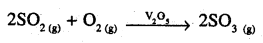Step (3)
SO3 produced is absorbed on H2SO4 to give H2S2O7(Oleum)

SO3+ H2SO4 → H2S2O7

This oleum is then diluted to obtain H2SO4, of the desired concentration. In practice, the plant is operated at 2 bar (pressure) and 720 K (temperature). The H2SO4 thus obtained is 96 – 98% pure.

Question 22.
How is SO2 an air pollutant?

• It combines with water vapour present in the atmosphere to form sulphuric acid. This causes acid rain. Acid rain damages soil, plants and buildings, especially those made of marble.
• Even in very low concentrations, SO2 causes irritation in the respiratory tract. It causes throat
and eye irritation and can also affect the larynx to cause breathlessness.
• It is extremely harmful to plants. Plants exposed to sulphur dioxide for a long time lose colour from their leaves. This condition is known as chlorosis. This happens because the formation of chlorophyll is affected by the presence of SO2.

Question 23.
Why are halogens strong oxidising agents?
The general electronic configuration of halogens is np5, where n = 2-6. Thus, halogens need only one more e- to complete their octet and to attain the stable noble gas configuration. Also, halogens are highly electronegative with low dissociation energies and high negative electron gain enthalpies. Therefore, they have a high tendency to gain an election. Hence, they act as strong oxidizing agents.Question 24.
Explain why fluorine forms only one
Fluorine forms only one oxoacid, i.e. HOF because of its high electronegativity and small size.

Question 25
Explain why inspite of nearly the same electronegativity, oxygen forms hydrogen bonding while chlorine does not.
Both chlorine and oxygen have almost the same electronegativity values, but chlorine rarely forms hydrogen bonding. This is because in comparison to chlorine, oxygen has a smaller size and as a result, a higher electron density per unit volume.

Question 26
Write two uses of ClO2.

1. It is used for purifying water
2. It is a used as a bleaching agent.

Question 27
Why are halogens coloured?
Almost all halogens are coloured. This is because halogens absorb radiations in the visible region. This results in the excitation of valence electrons to a higher energy region. Since the amount of energy required for excitation differ for each halogen, each halogen displays a colour.

Question 28
Write the reactions of F2 and Cl2 with water.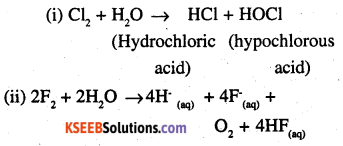Question 29
How can you prepare Cl, from HCl and HCl from Cl2? Write reactions only.
Cl2 can be prepared from HC1 by Deacon’s process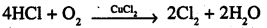(ii) HCl can be prepared from Cl by treating it with water
Cl2 + H2O → HCl + HOCl

Question 30
What inspired N. Bartlett for carrying out reaction between Xe and PtF6? ‘
Neil Bartlett initially carried out a reaction between oxygen and PtFg. This resulted in the formation of a red compound, O+2 [Pt F6 ].

Later he realised that the first ionization energy of oxygen (1175 kJ/mol) and Xe (1170 kJ/mol) is almost the same. Thus, he tried to prepare a compound with Xe and PtF6. He was successful and a red coloured compound, Xe+ [PtF6]- was formed.

Question 31.
What are the oxidation states of phosphorus in the following:
(i) H3PO3
(ii) PCl3
(iii) Ca3P2
(iv) Na3PO4
(v) POF3?
Let oxidation state of P be x
(i) H3PO3
3 + x + 3 (-2) = 0
3 + x – 6 = 0
x = 3.

(ii) PCl3
x + 3 (-1) = 0
x – 3 = 0
x = 3

(iii) Ca3P2
3(2) + 2x = 0
6 + 2x = 0
x = -3

(iv) Na3PO4
3 x 1 +x + 4(-2) = 0
3 + x – 8 = 0
x = 5

(v) POF3
x + (-2) + 3x (-1) = 0
x – 5 = 0
x = 5.

Question 32.
Write balanced equations for the following:
(i) NaCl is heated with sulphuric acid in the presence of MnO2.
(ii) Chlorine gas is passed into a solution of Nal in water.
(i) 4NaCl + MnO2 + 4H2SO4 → MnCl2 + 4NaHSO4+ 2H2O + Cl2
(ii) Cl2 + Nal → 2NaCl + I2Question 33.
How are xenon fluorides XeF2, XeF4 and XeF. obtained?
XeF2, X3F4 and XeF6 are obtained by a direct reaction between Xe and F2. The condition under which the reaction is carried out determines the product.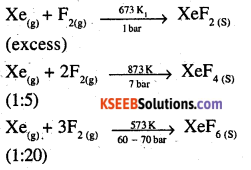Question 34
With what neutral molecule’ is ClO isoelectronic? Is that molecule a Lewis base?
ClO is isoelectronic to ClF. Also, both species contain 26es in all as shown.
Total electrons in ClO = 17 + 8 + 1 = 26
In ClF =17 + 9 =26
ClF, acts like a lewis base as it accepts e-s from F to form ClF3

Question 35
How are XeO3 and XeOF4 prepared?
(i) XeO3 can be prepared in two ways as shown:
6XeF4 + 12H2O → 4Xe + 2XeO3 + 24HF + 3O2 XeF6 + 3H2O → XeO3 + 6HF
(ii) XeF4 can be prepared using XeF6
XeF6+ H2O → XeOF4 + 2HF

Question 36
Arrange the following in the order of property indicated for each set:
(i) F2, Cl2, Br2, I2 – increasing bond dissociation enthalpy.
Bond dissociation energy usually decreases on moving down a group as the atomic size increases. However, the bond dissociation energy of F2 is lower than that of Cl2 and Br2. This is due to the small atomic size of fluorine, Thus, the increasing order for bond dissociation energy among halogens is as follows:

I2 < F2 < Br2 < Cl2

(ii) HF, HCl, HBr, HI – increasing acid strength.
HF < HCl < HBr < HI
The bond dissociation energy of HX molecules where X – F, Cl, Br, I, decreases with an increase in the atomic size. Since H – I bond is the weakest, HI is the strongest acid.

(iii) NH3, PH3, ASH3, SbH3, BiH3 – increasing base strength.
BiH3 < SbH3 < AsH3 < PH3 < NH3 On moving from nitrogen to bismuth, the size of the atom increases while the electron density on the atom decreases. Thus, the basic strength decreases.Question 37.
Which one of the following does not exist?
(i) XeOF4
(ii) NeF2
(iii) XeF2
(iv) XeF
(ii) NeF2

Question 38.
Give the formula and describe the structure of a noble gas species which is isostructural with:
(i) ICl4
(ii) IBr2
(iii) BrO3
(i) XeF4 is isoelectronic with ICl4 and has square planar geometry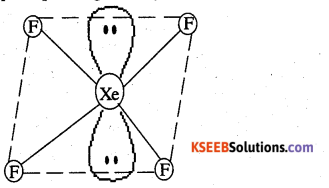(ii) XeF2 is isoelectronic to IBr2 and has a linear structure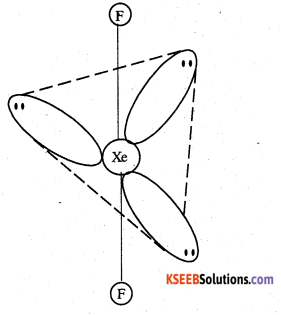(iii) XeO3 is isostructural to BrO3 and has a pyramidal molecular structure.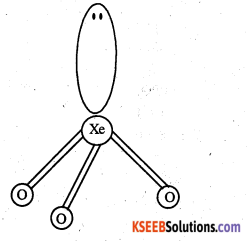Question 39.
Why do noble gases have comparatively large atomic sizes?
Noble gases do not form molecules. In case of noble gases. the atomic radii corresponds to Van der Waa1s radii. On the other hand, the atomic radii of other elements correspond to their covalent radii. By definition, Yan der WaaI’s radii are larger than covalent radii. It is for this reason that noble gases are very large in size as compared to other atoms belonging to the same period.Question 40
List the uses of neon and argon gases.
Uses of neon gas:
(i) It is mixed with helium to protect electrical equipments from high voltage.
(ii) It is filled in discharge tubes with characteristic colours.
(iii) It is used in beacon lights.

Uses of argon gas:
(i) Argon along with nitrogen is used in gas filled electric lamps. This is because Ar is more inert than N.
(ii) It is usually used to provide an inert temperature in a high metallurgical process.
(iii) It is also used in laboratories to handle air sensitive substances.

Question 1.
What is the electronic .configuration of p-block elements?
ns2np0 – 5

Question 2.
Write three uses of nitrogen?

1. Used in the manufacture of nitric acid, ammonia, calcium cyanamide.
2. Inert atmosphere for iron and steel industry.
3. It is used as a refrigerant.

Question 3.
Write three physical properties of Ammonia?

1. It is a colourless gas.
2. It is lighter than air.
3. Extremely soluble in water.

Question 4.
Write three physical properties of Nitric acid?

1. Colourless fuming liquid.
2. Melting point is 231.4k.
3. Boiling point is 355.6 k.

Question 5.
What are the three allotropic forms of phosphorous.

1.  white phosphorous
2. Red phosphorous
3. Black phosphorous.

Question 6.
Write the chemical formula of Hypo phosphorus acid.
H3PO2.Question 7.
Write the chemical formula of orthophosphorous acid.
H3PO3

Question 8.
Write the chemical formula of pyrophosphorous acid.